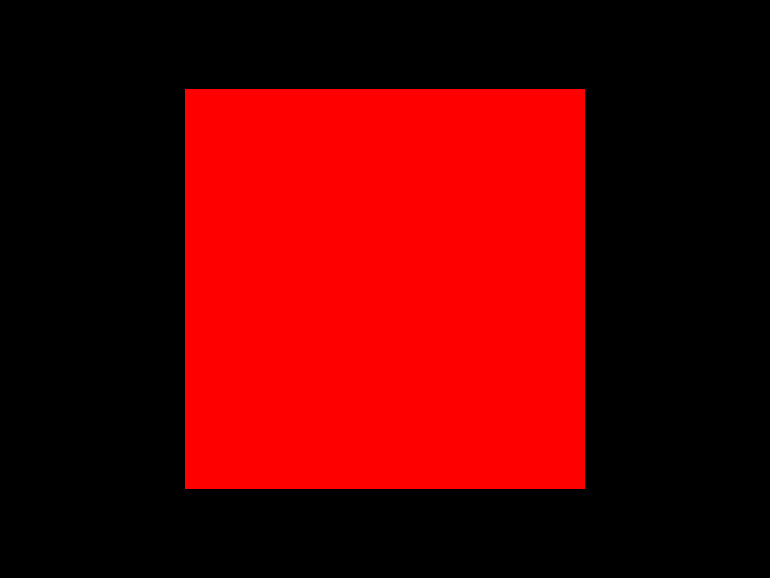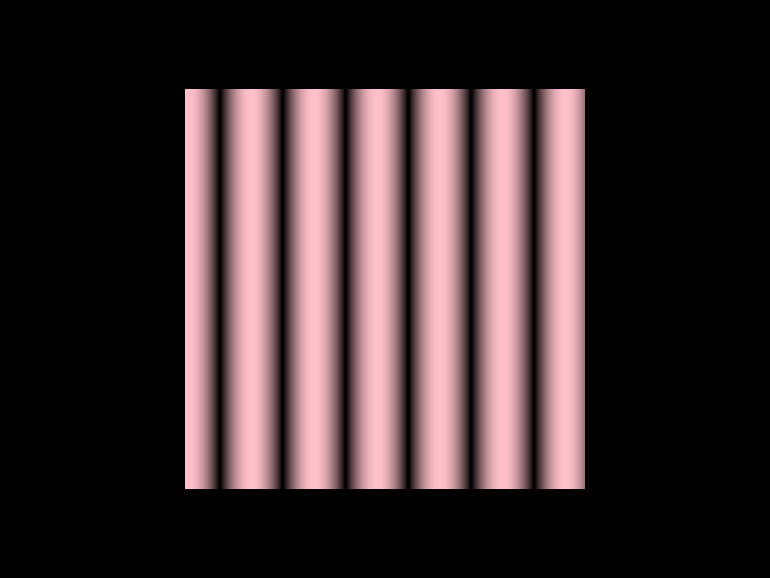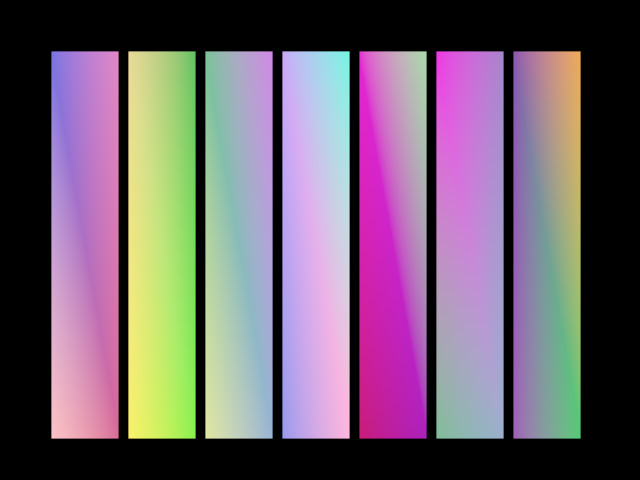Shade styles are used to change the drawing behaviour of the `Drawer` affecting the appearance of all drawing primitives.

Shade styles are composed of two types of transforms: vertex transforms and fragment transforms. The two transforms are applied in separate stages of the rendering process. In the vertex transform it is possible to change the geometry of what is drawn, and in the fragment transform it is possible to change the appearance of that geometry. A shade style can affect vertices, fragments or both.

A selection of preset ready-to-use shade styles is provided by `orx-shade-styles`

For those interested in authoring shade styles it is helpful to have some basic understanding of shaders and GLSL.

## Basic usage

In essence shade styles are fragments of GLSL code that are inserted into OPENRNDRs templated shaders.

As a quick first step we override the output to red in the following snippet``````fun main() = application {
program {
extend {
fragmentTransform = "x_fill.rgb = vec3(1.0, 0.0, 0.0);"
}
drawer.fill = ColorRGBa.PINK
drawer.stroke = null
drawer.rectangle(width / 2.0 - 200.0, height / 2.0 - 200.0, 400.0, 400.00)
}
}
}
``````

The idea of shade styles is to allow more complex changes in the appearance. In the next snippet we create a wavy pattern by using cosines and the screen position.``````fun main() = application {
program {
extend {
fragmentTransform = """
float c = cos(c_screenPosition.x * 0.1) * 0.5 + 0.5;
x_fill.rgb *= vec3(c, c, c);
""".trimMargin()
}
drawer.fill = ColorRGBa.PINK
drawer.stroke = null
drawer.rectangle(width / 2.0 - 200.0, height / 2.0 - 200.0, 400.0, 400.00)
}
}
}
``````

In the next step we introduce animation by adding an external clock signal to the shade style. Shade styles have parameters that can be used for this.

Notice how parameters like `time` receive a `p_` prefix in the GLSL world. This makes it easy to distinguish the uniforms we send into shaders from other variables declared by the framework.

``````fun main() = application {
program {
extend {
fragmentTransform = """
float c = cos(c_screenPosition.x * 0.1 + p_time) * 0.5 + 0.5;
x_fill.rgb *= vec3(c, c, c);
""".trimMargin()
parameter("time", seconds)
}
drawer.fill = ColorRGBa.PINK
drawer.stroke = null
drawer.rectangle(width / 2.0 - 200.0, height / 2.0 - 200.0, 400.0, 400.0)
}
}
}
``````

## Usage examples

Here follow some examples of common problems that are solved using shade styles.

### Mapping images on shapes

``````fun main() = application {
program {
image.filter(MinifyingFilter.LINEAR_MIPMAP_NEAREST, MagnifyingFilter.LINEAR)
extend {
fragmentTransform = """
vec2 texCoord = c_boundsPosition.xy;
texCoord.y = 1.0 - texCoord.y;
vec2 size = textureSize(p_image, 0);
texCoord.x /= size.x/size.y;
x_fill = texture(p_image, texCoord);
"""
parameter("image", image)
}

val shape = Circle(width / 2.0, height / 2.0, 110.0).shape
drawer.translate(cos(seconds) * 100.0, sin(seconds) * 100.0)
drawer.shape(shape)
}
}
}
``````

### 3D mesh distortion

This example shows that one can also modify the vertex shader, in this case to displace vertices using sine functions. The current time in seconds is passed into the shader to produce a wavy effect. The fragment shader uses sine functions to specify colors depending on the world position of each vertex.

``````fun main() = application {
program {
val sphere = sphereMesh(32, 32, 0.6)
vertexTransform = """
vec3 p = x_position * 8.0 + p_seconds;
// displace the vertices
x_position.x += sin(p.y) * 0.1;
x_position.y += sin(p.z) * 0.1;
x_position.z += sin(p.x) * 0.1;
""".trimIndent()

fragmentTransform = """
vec3 c = sin(v_worldPosition) * 0.5 + 0.5;
x_fill = vec4(c, 1.0);
""".trimIndent()
}

val camera = OrbitalCamera(Vector3.UNIT_Z, Vector3.ZERO)

extend(camera)
extend {
camera.rotate(0.2, 0.0)
style.parameter("seconds", seconds)
drawer.vertexBuffer(sphere, DrawPrimitive.LINES)
}
}
}
``````

### Prefix overview

Listed below is an overview of all the prefixes used in the shade style language.

prefix Scope Description
`u_` all system uniforms passed in from Drawer
`a_` vertex transform vertex attribute
`va_` fragment transform varying attribute, interpolation passed from vertex to fragment shader
`v_` fragment transform varying values, interpolation passed from vertex to fragment shader
`i_` vertex transform instance attribute
`vi_` fragment transform varying instance attribute
`x_` all transformable value
`p_` all user provided value
`o_` fragment transform output value (always `vec4`)
`d_` all shader definitions

### Standard uniforms

Listed below is an overview of all the prefixes used in the shade style language.

Uniform name GLSL type Description
`u_modelNormalMatrix` mat4 matrix used to transform vertex normals from object to world space
`u_modelMatrix` mat4 matrix used to transform vertices from object to world space
`u_viewNormalMatrix` mat4 matrix used to transform vertex normals from world space to view space
`u_viewMatrix` mat4 matrix used to transform vertices from world space to view space
`u_projectionMatrix` mat4 matrix used to transform vertices from view space to clip space
`u_contentScale` float the active content scale
`u_viewDimensions` vec2 the dimensions of the target viewport
`u_fill` vec4 the Drawer fill color
`u_stroke` vec4 the Drawer stroke color
`u_strokeWeight` float the Drawer strokeWeight
`u_colorMatrix` float the Drawer color matrix

### Standard Attributes

Attributes are only directly accessible in the vertex transform. However interpolated forms of the the attributes are passed to the fragment transform.

Attribute name GLSL type Description
`a_position` vec3 the position
`a_normal` vec3 the normal
`a_color` vec3 the color

The interpolated versions that are only accessible in the fragment transform.

Attribute name GLSL type Description
`va_position` vec3 the interpolated position
`va_normal` vec3 the interpolated normal
`va_color` vec3 the interpolated color

### Other interpolated values

These values are calculated in the vertex shader and only accessible in the fragment transform.

Value name GLSL type Description
`v_worldNormal` vec3 interpolated normal in world coordinates
`v_viewNormal` vec3 interpolated normal in view coordinates
`v_worldPosition` vec3 interpolated position in world coordinates
`v_viewPosition` vec3 interpolated position in view coordinates
`v_clipPosition` vec4 interpolated position in clip coordinates
`v_modelNormalMatrix` mat4 non-interpolated (flat) model normal matrix

### Transformable values

These are values that can be transformed using shade styles.

#### Vertex transform

Variable name GLSL type Description
`x_position` `vec3` vertex position, initialized with value `a_position`
`x_normal` `vec3` vertex normal, initialized with value `a_normal`
`x_viewMatrix` `mat4` view matrix
`x_normalMatrix` `mat4` normal matrix, initialized with `normalMatrix`
`x_projectionMatrix` `mat4` projection matrix, initialized with `projectionMatrix`
`x_modelMatrix` `mat4` model matrix, initialized with `modelMatrix`
`x_modelNormalMatrix` `mat4` model normal matrix, initialized with `modelNormalMatrix`
`x_viewNormalMatrix` `mat4` view normal matrix, initialized with `viewNormalMatrix`

#### Fragment transform

Variable name GLSL type Description
`x_fill` `vec4` The fill color written to the fragment
`x_stroke` `vec4` The stroke color written to the fragment

### Constants

Constant name Scope GLSL type Description
`c_element` all int the element index in batched rendering
`c_instance` all int the instance index in instanced rendering
`c_screenPosition` fragment transform vec2 the position on screen in device coordinates
`c_contourPosition` fragment transform float the position on the contour, between 0.0 and contour.length. Only non-zero when drawing line segments and contours
`c_boundsPosition` fragment transform vec3 the bounding box position of the current shape or contour stored in `.xy`
`c_boundsSize` fragment transform vec3 the bounding box size of the current shape or contour stored in `.xy`

### Parameters

Parameters can be used to supply external data to transforms. Parameters are translated to shader uniforms and are exposed by uniforms with the `p_` prefix.

#### ColorBuffer parameters

Can be used to map images.

#### BufferTexture parameters

Can be used to map custom values.

#### Supported parameter types:

JVM type GLSL type
`float` `float`
`Vector2` `vec2`
`Vector3` `vec3`
`Vector4` `vec4`
`ColorRGBa` `vec4`
`Matrix44` `mat4`
`DepthBuffer` `sampler2D`
`ColorBuffer` `sampler2D`
`BufferTexture` `samplerBuffer`

#### Source code

One can explore the source code to find out how attributes and uniforms are used:

## Vertex and fragment preambles

Since the code declared in `vertexTransform` and `fragmentTransform` end up inside the `main()` function of shader programs, you may be wondering how you could declare custom functions, for example, to calculate a noise value, a random value or to do matrix rotations (common functions used in shader programs).

To achieve this, we use two keywords: `vertexPreamble` and `fragmentPreamble`. The code found in these strings gets inserted into the shader programs before the `main()` function, allowing us to declare custom functions or even varyings (to pass values from the vertex shader into the fragment shader).``````fun main() = application {
program {
// Define the `random` function and declare a `c`
// variable to pass to the fragment shader.
vertexPreamble = """
float random(vec2 st) {
return fract(sin(dot(st.xy,
vec2(12.9898, 78.233))) * 43758.5453123);
}
out vec3 c;
""".trimIndent()

// Calculate the value of `c` per vertex.
// It will get interpolated by the GPU.
vertexTransform = """
c.r = random(x_position.xy);
c.g = random(x_position.yx);
c.b = random(x_position.xy + 1.0);
""".trimIndent()

fragmentPreamble = "in vec3 c;"
// Use the value of `c` to set the color of a pixel.
fragmentTransform = "x_fill.rgb = c;"
}
extend {
repeat(7) {
// Notice how we do not set `drawer.fill`.
drawer.rectangle(50.0 + it * 77, 50.0, 70.0, 390.0)
}
}
}
}
``````

If you want to study the vertex or the fragment shader in their final form, a simple technique is to provoke an error. Throw in some incorrect syntax: for example, add an `x` character at the beginning of the vertex or the fragment shader.
When you try to run the program, it will fail, and a file called `ShaderError.glsl` will be written into the project’s root. Study that file to understand what attributes and uniforms are available and to figure out why your program fails in case the syntax error was not intentional.